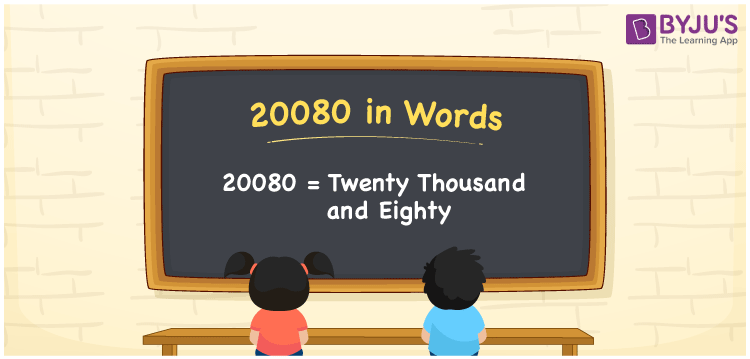# 20080 in Words

20080 in words is written as Twenty thousand eighty. In both the International System of Numerals and the Indian System of Numerals, 20080 in words is written as Twenty thousand eighty. The number 20080 is a Cardinal Number as it represents some quantity. For example, “she has 20080 rupees in her bank account”.

 20080 in Words Twenty thousand eighty Twenty thousand eighty in number 20080

## 20080 in English Words

20080 in English words is read as “Twenty thousand eighty”.## How to Write 20080 in Words?

To write 20080 in words, we shall use the place value chart. In the place value chart, put 2 in the ten thousands, 0 in the thousands, hundreds, and ones, and 8 in the tens, respectively. Let us make a place value chart to write the number 20080 in words.

 Ten Thousands Thousands Hundreds Tens Ones 2 0 0 8 0

Thus, we can write the expanded form as

2 × Ten Thousand + 0 × Thousand + 0 × Hundred + 8 × Ten + 0 × One

= 2 × 10000 + 0 × 1000 + 0 × 100 + 8 × 10 + 0 × 1

= 20000 + 0 + 0 + 80 + 0

= 20080

= Twenty thousand eighty.

20080 is a natural number that lies between 20079 and 20081.

20080 in words – Twenty thousand eighty

• Is 20080 an odd number? – No
• Is 20080 an even number? – Yes
• Is 20080 a perfect square number? – No
• Is 20080 a perfect cube number? – No
• Is 20080 a prime number? – No
• Is 20080 a composite number? – Yes

## Frequently Asked Questions on 20080 in Words

Q1

### How to write 20080 in words?

20080 in words is written as Twenty thousand eighty.
Q2

### How to write 20080 in the International and Indian System of Numerals?

In both, the system of numerals, 20080 in words, is written as Twenty thousand eighty.
Q3

### How to write 20080 in a place value chart?

In the place value chart, write 2 in the ten thousands, 0 in the thousands, hundreds, and ones, and 8 in the tens, respectively.